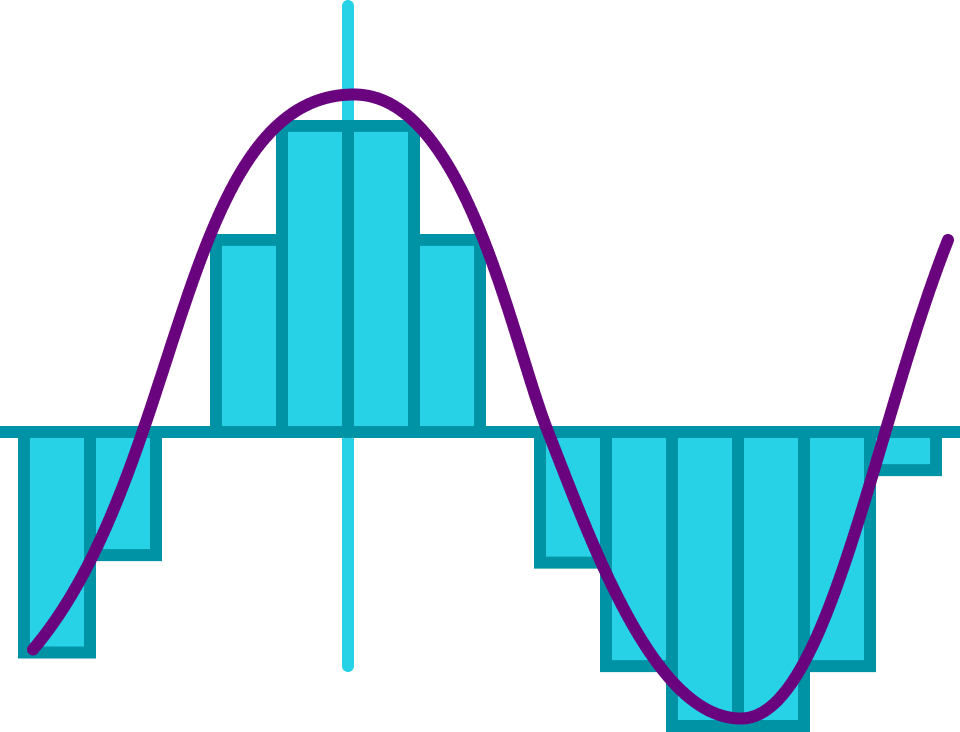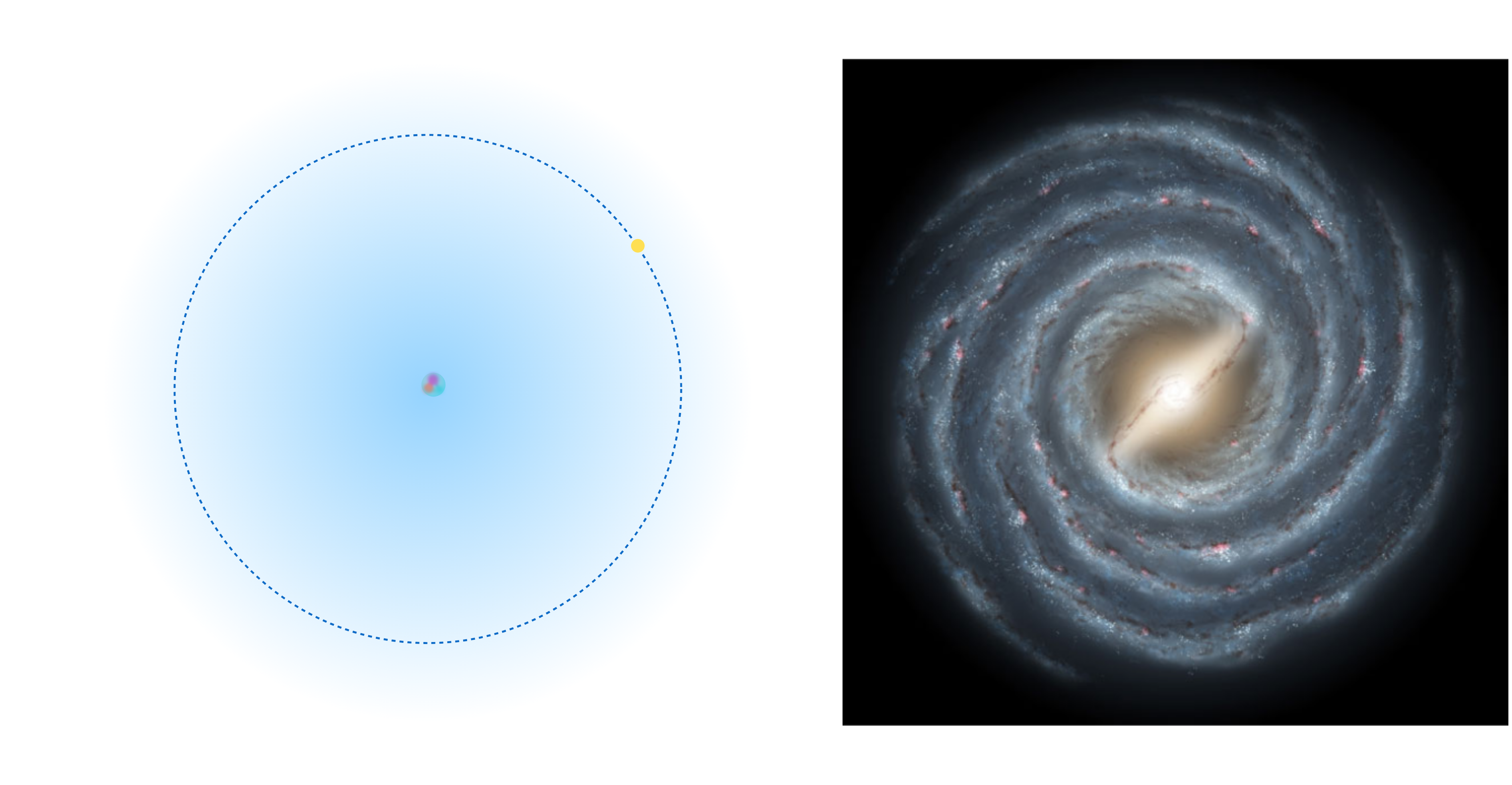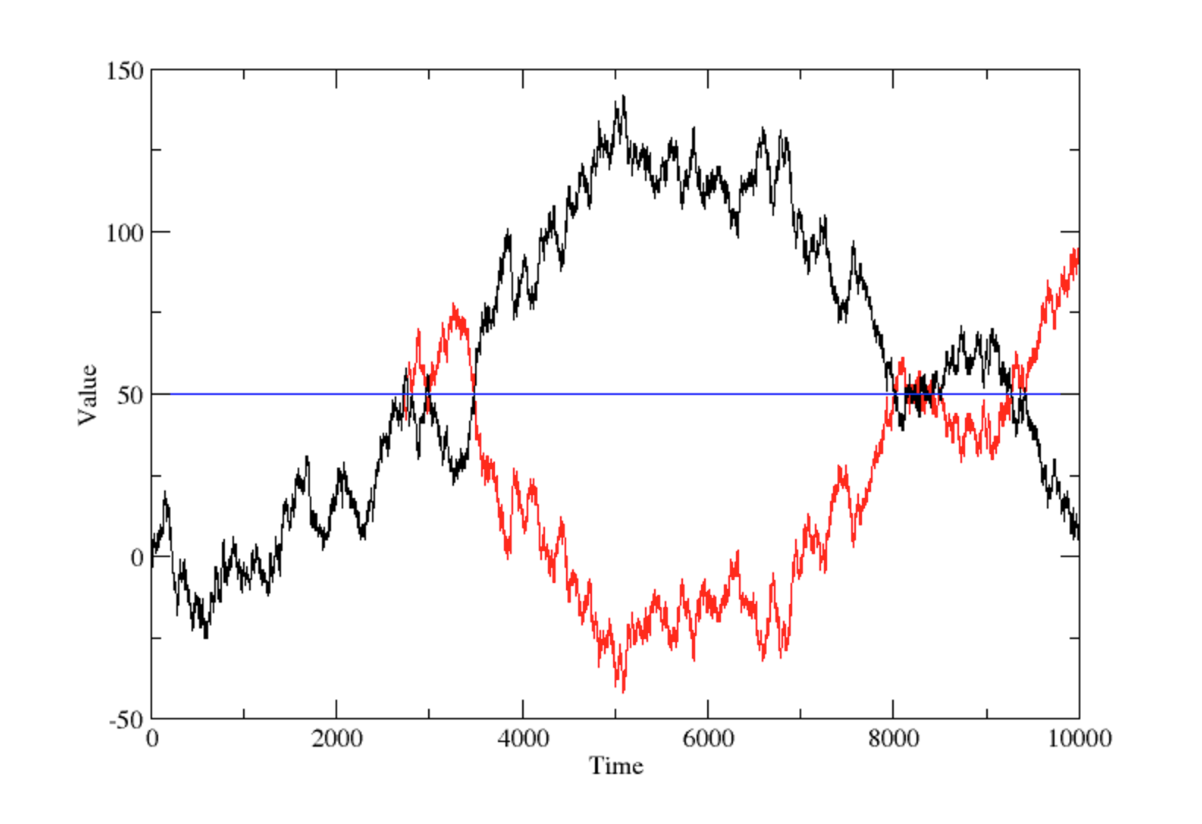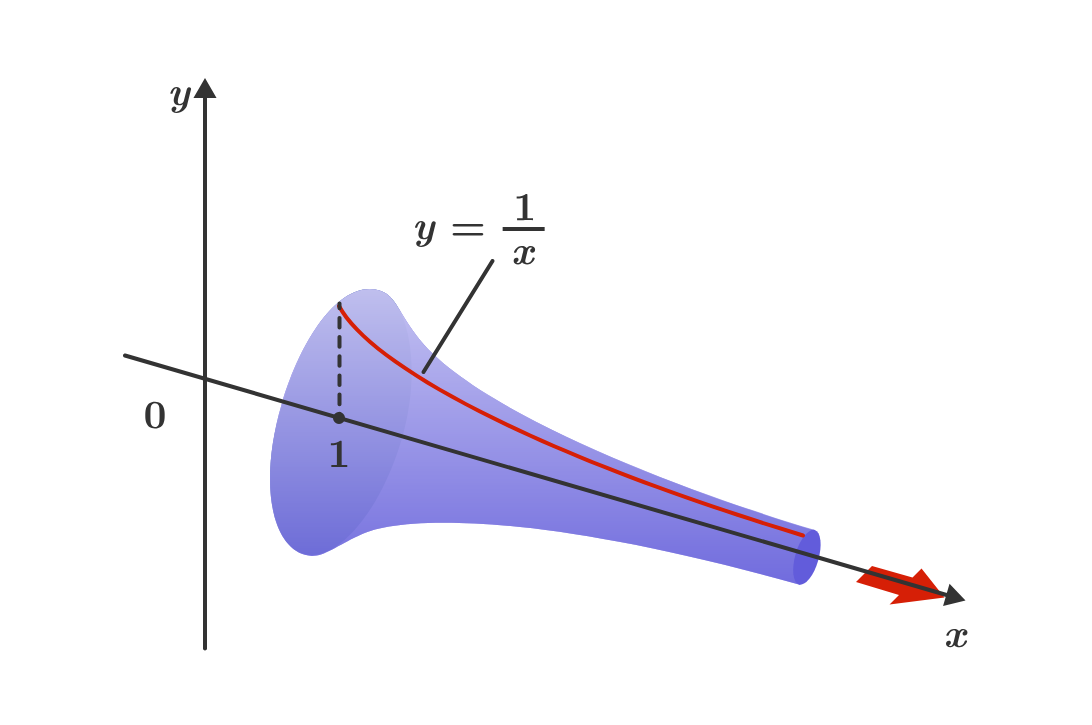# Calculus in a Nutshell

Calculus is everywhere, and the rewards for learning it are immense. Physicists use calculus to understand the world from the subatomic level to the far reaches of the cosmos.Financial analysts use calculus to model stock market fluctuations.Without calculus, engineers couldn't make skyscrapers, airplanes, or the computing device you are using right now to view this course.## Calculus in a Nutshell

### Introduction to Calculus

# Calculus in a Nutshell

We're about to start a grand journey. Like all mathematics, calculus is not a spectator sport. Here, you'll engage with challenging problems and interactive visualizations designed to guide you through the essential ideas of calculus. You'll be introduced to the most important theorems underlying the subject and see some of the many applications of calculus to the real world.

This unit will give you a glimpse of the shape of things to come. Let's start our whirlwind tour of some of the most exciting elements this course has to offer!

## Calculus in a Nutshell

### Introduction to Calculus

# Calculus in a Nutshell

In a nutshell, calculus is the mathematics of limits. We'll define what limits are soon, but first, we're going to sharpen your instincts and strengthen your intuition by exploring a few problems where the application of limits is intuitive.

In the scenarios in this unit, you'll learn why limits are powerful tools for making strong predictions.

As a first example, consider the function modeling the erratic pig below. Under what circumstances can we make a strong prediction about the end of the pig's run when it approaches the fence?

## Calculus in a Nutshell

### Introduction to Calculus

# Calculus in a Nutshell

Some of our visualizations, like the runaway pig, are cartoons capturing abstract ideas. Others, like the one below, are designed to help illustrate problem-solving skills. The visualization below demonstrates how:

“As $$x$$ gets very, very large, the values of $$1 + e^{-x}$$ become very close to $$\text{__________}."$$

What number completes this statement?

Notice that the slider below the graph lets you control the value of $$\epsilon$$ and the green-shaded area $$(x \geq M)$$ is the range of $$x$$ for which the value of function is between $$1+\epsilon$$ and $$1 - \epsilon .$$

## Calculus in a Nutshell

### Introduction to Calculus

# Calculus in a Nutshell

One of the most important uses of the limit is in defining the derivative, a very powerful tool in physics and engineering. The derivative measures how fast a function changes. With limits, we can define how to take the derivative of a function formally, but for now, let's just explore a few examples!

In the visualization below, as you control the value of $$a$$, the function $$f(x) = x^{a}$$ will display on the left, and the derivative of that function will display on the right. Play around with the controls and see if you can see a pattern in the relationship between the original graphs and their derivatives.

When you are done experimenting, complete the following statement:

If $$f(x) = x^{a}$$, then the derivative seems proportional to $$\text{__________}.$$

By the end of Chapter 5, we'll not only know how to find derivatives, but we'll understand why this observation is true.

## Calculus in a Nutshell

### Introduction to Calculus

# Calculus in a Nutshell

We can also define integrals with limits. Like derivatives, integrals allow us to compute many interesting things, like the volume of a geometric shape, the center of mass of a solid physical object, and the probability of observing a random event.

The applications of integrals are limitless, and we'll learn how to compute them in Chapters 6-9. To whet your appetite, take a look at the shape below.The shape is called Gabriel's horn. It has the strange property that you can fill it with a few gallons of paint, but you can never finish painting the outside, no matter how much paint you have! (Mathematically, this means it has infinite surface area but finite volume.)

We'll find out why this is so in Chapter 8.

## Calculus in a Nutshell

### Introduction to Calculus

# Calculus in a Nutshell

The penultimate chapter deals with infinite series, a tool which will allow us to solve complex, real-world differential equations, and even helped kick off the quantum revolution at the dawn of the twentieth century.

One of our specific goals in the last chapter will be to show why Euler's formula $e^{i \theta} = \cos( \theta) + i \sin(\theta)$ is true. One special case of this equation has even been called “the most beautiful equation in all of mathematics,” since it relates the five most important numbers $$0, 1, i = \sqrt{-1} , \pi,$$ and $$e$$ together in one elegant formula: $e^{i \pi} + 1 = 0 .$

## Calculus in a Nutshell

### Introduction to Calculus

# Calculus in a Nutshell

The slider below helps us understand the Tower of Lire puzzle we'll solve towards the end of the course. Is there any way to stack the blocks to make the top block of the tower reach past the dotted line while keeping the position of the center of mass of the tower (indicated by the circle on the horizontal axis) left of the edge of the ledge?

The circle on the horizontal axis indicates the location of the center of mass of the whole block tower. In real life, if the center of mass of the tower moves anywhere to the right of the ledge's edge, the tower will fall off the ledge. In this visualization, the blocks won't fall, but the circle on the axis will turn red if the center of mass is past the edge of the ledge.

## Calculus in a Nutshell

### Introduction to Calculus

# Calculus in a Nutshell

We have our work cut out for us. The journey is challenging, but the rewards are plentiful. The rest of this introductory chapter will ease us into some of the most important ideas of calculus.

The next unit introduces one of the most enjoyable applications of calculus: resolving Zeno's paradox of motion. Our exploration will be largely visual with some mathematical exercises sprinkled in.

## Calculus in a Nutshell

### Introduction to Calculus

×

Problem Loading...

Note Loading...

Set Loading...